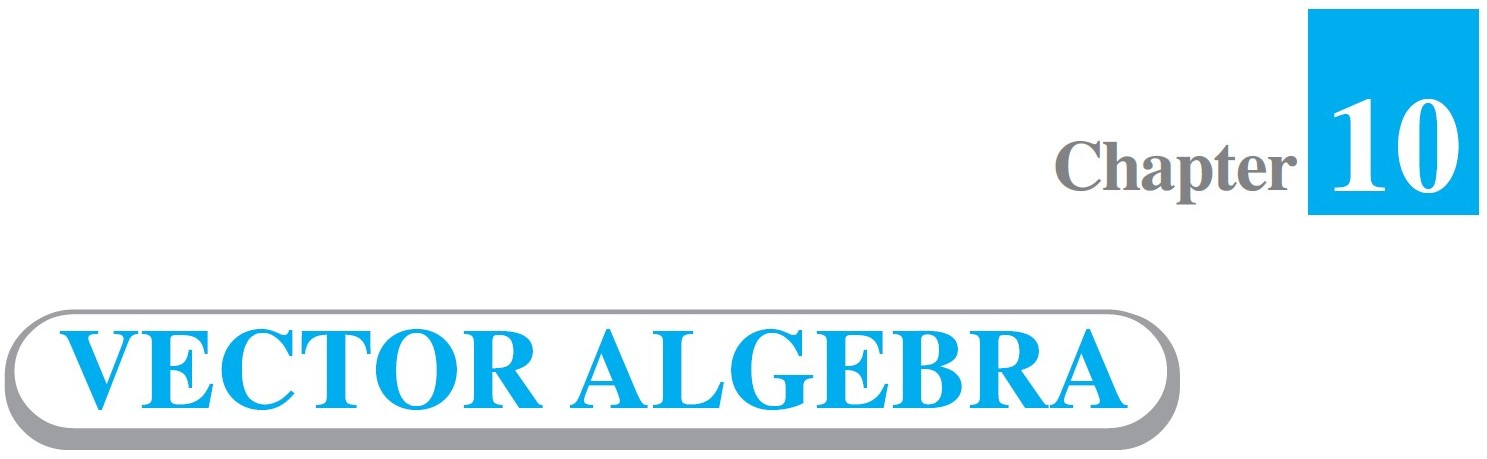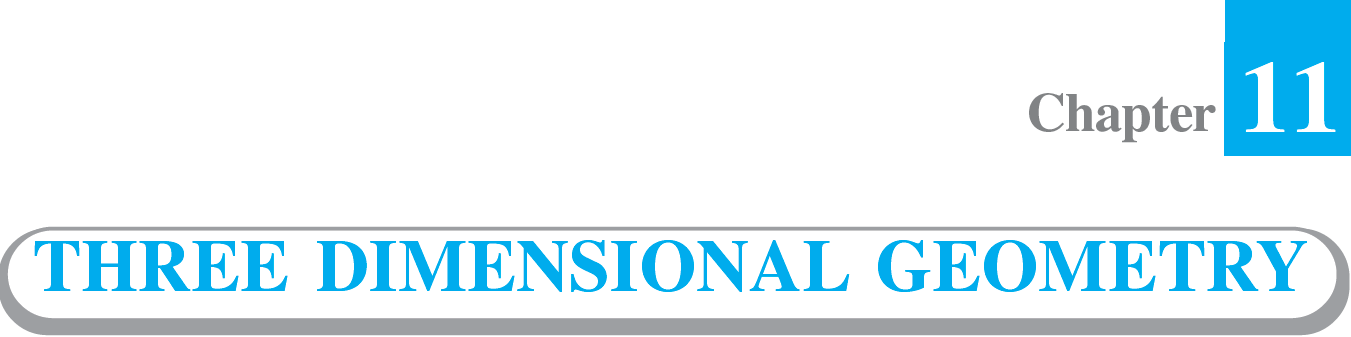# NCERT Solutions for Class 12 Maths

Ashish Kumar – Let’s Learn is unified online learning platform that provides NCERT Solutions for Class 12 Maths with online animated video lectures, pdf notes and assignments.

We provide perfect opportunity for students to prepare for their class 12 maths boards and entrances as we had only one aim in our mind and that is to provide excellent education with deep learning resources to every student who can or cannot afford thousands of rupees to attend a coaching class or hire special educator for their entrances and Board Exams.

## Select ChapterTypes of relations: Reflexive, symmetric, transitive and equivalence relations. One to one and onto functions, composite functions, inverse of a function. Binary operations.Definition, range, domain, principal value branches. Graphs of inverse trigonometric functions. Elementary properties of inverse trigonometric functions.Concept, notation, order, equality, types of matrices, zero matrix, transpose of a matrix, symmetric and skew symmetric matrices. Addition, multiplication and scalar multiplication of matrices, simple properties of addition, multiplication and scalar multiplication. Non-commutativity of multiplication of matrices and existence of non-zero matrices whose product is the zero matrix (restrict to square matrices of order 2). Concept of elementary row and column operations. Invertible matrices and proof of the uniqueness of inverse, if it exists; (Here all matrices will have real entries).Determinant of a square matrix (up to 3 × 3 matrices), properties of determinants, minors, cofactors and applications of determinants in finding the area of a triangle. Adjoint and inverse of a square matrix. Consistency, inconsistency and number of solutions of system of linear equations by examples, solving system of linear equations in two or three variables (having unique solution) using inverse of a matrix.Continuity and differentiability, derivative of composite functions, chain rule, derivatives of inverse trigonometric functions, derivative of implicit function. Concepts of exponential, logarithmic functions. Logarithmic differentiation. Derivative of functions expressed in parametric
forms. Second order derivatives. Rolle’s and Lagrange’s Mean Value Theorems (without proof) and their geometric interpretations.Applications of derivatives: Rate of change, increasing/decreasing functions, tangents and normals, approximation, maxima and minima (first derivative test motivated geometrically and second derivative test given as a provable tool). Simple problems (that illustrate basic principles and understanding of the subject as well as real-life situations).Integration as inverse process of differentiation. Integration of a variety of functions by substitution, by partial fractions and by parts, only simple integrals of the type –

\int{\frac{dx}{x^2 \pm a^2}}

\int{\frac{dx}{\sqrt{x^2 \pm a^2}}}

\int{\frac{dx}{a^2 – x^2}}

\int{\frac{dx}{ax^2 +bx + c}}

\int{\frac{dx}{\sqrt{ax^2 +bx + c}}}

\int{\frac{px + q}{ax^2 +bx + c}}  dx

\int{\frac{px + q}{\sqrt{ax^2 +bx + c}}}  dx

\int{\sqrt{a^2 \pm x^2}} dx

\int{\sqrt{x^2 – a^2}} dx

\int{\sqrt{ax^2+bx+c}} dx

\int{(px + q){\sqrt{ax^2+bx+c}}} dx

to be evaluated.
Definite integrals as a limit of a sum. Fundamental Theorem of Calculus (without proof). Basic properties of definite integrals and evaluation of definite integrals.Vectors and scalars, magnitude and direction of a vector. Direction cosines/ratios of vectors. Types of vectors (equal, unit, zero, parallel and collinear vectors), position vector of a point, negative of a vector, components of a vector, addition of vectors, multiplication of a vector by a scalar, position vector of a point dividing a line segment in a given ratio. Scalar (dot) product of vectors, projection of a vector on a line. Vector (cross) product of vectors, scalar triple product.Direction cosines and direction ratios of a line joining two points. Cartesian and vector equation of a line, coplanar and skew lines, shortest distance between two lines. Cartesian and vector equation of a plane. Angle between (i) two lines, (ii) two planes, (iii) a line and a plane. Distance of a point from a plane.